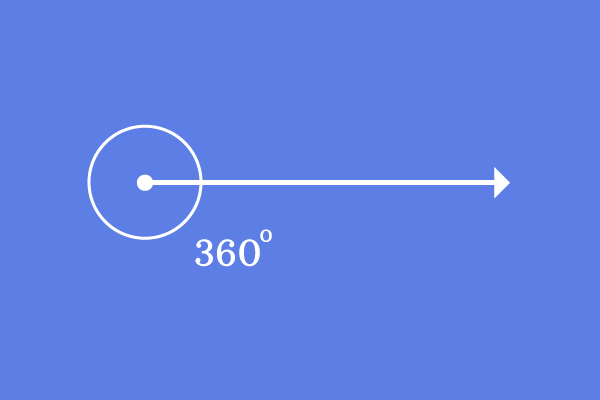# Complete angle

## Definition

An angle of $360^\circ$ is called a complete angle.A straight line makes an angle of $360^\circ$ to reach its initial position completely by the rotation. Hence, the angle is called as the complete angle.

A complete angle is represented same as the zero angle but there is one difference between them and it is the amount of rotation.

### Representation

The complete angle is represented in three different angle measuring systems.

1. It is denoted by $360^°$ in Sexagesimal system
2. It is denoted by $2\pi$ in Circular system
3. It is denoted by $400^g$ in Centesimal system

#### Formation

There are two possibilities to form complete angles in geometric system.

1
##### Complete angle by a Line$\overrightarrow{CD}$ is a ray and it is initially at a position on the plane.

The ray $\overrightarrow{CD}$ is rotated an angle of $360^\circ$ to reach the same position where the same ray is called as $\overrightarrow{CE}$.

The angle made by the ray to reach its final position from its initial position is $\angle ECD$ and the amount of rotation is $360^\circ$.

$\angle ECD = 360^\circ$

The angle $ECD$ is $360^\circ$. So, the angle $ECD$ is an example for the complete angle.

2
##### Complete angle between two lines$\overrightarrow{FG}$ is a ray and $\overrightarrow{FH}$ is another ray. The two rays make same angle but the angle between them is a complete angle.

The angle between them is denoted by $\angle GFH$.

$\angle GFH = 360^\circ$

Therefore, the angle $GFH$ is another example of a complete angle.

Latest Math Topics
Jun 26, 2023
Jun 23, 2023

Latest Math Problems
Jul 01, 2023
Jun 25, 2023
###### Math Questions

The math problems with solutions to learn how to solve a problem.

Learn solutions

Practice now

###### Math Videos

The math videos tutorials with visual graphics to learn every concept.

Watch now

###### Subscribe us

Get the latest math updates from the Math Doubts by subscribing us.